## Nuclear physics and neutron-rich nucleosynthesisLA-UR-18-XXXXX

### Matthew Mumpower

ANL Seminar

Monday Nov. 12$^{th}$ 2018FIRE Collaboration
Fission In R-process Elements

## Neutron stars

Mass of around 1.1 to 3 M$_\odot$

Density $10^{9}$ to $10^{17}$ kg/m$^{3}$

Magnetic field $10^{10}$ Tesla

Color depends on equation of state...

Ozel & Freire ARA&A 54 401 (2016) • Figure by Mumpower

## A close binary of neutron stars

GW170817 - named for the day it was discovered

NGC 4993 - about 40 Mpc away

Abbott et al. PRL 119 16 (2017) • Im et al. ApJL 849 1 (2017) • Figure by Mumpower

## A close binary of neutron stars

Important for GCE

Coalescence time: ~1 million years

Cote et al. ApJ 855 2 (2018) • Figure by Mumpower

## Grav waves are emitted

Chirp mass $\sim 1.188$
implies NS binary

Abbott et al. PRL 119 16 (2017) • Smartt et al. Nature 551 75 (2017) • Figure by Mumpower

## Neutron stars merge

Rosswog Nature 500 535 (2013) • Figure by Mumpower

## Many open problems...

Is GW170817 a typical event?

What is the morphology of the remnant?

What is the typical timescale for the merger? (statistics)

What are the properties of the equation of state?

What is the tidal deformability?

What role can neutrinos play?

How much material can be ejected?

What heavy elements can be created?

Figure by Mumpower

## Electromagnetic counterpartModel assumptions: M$_{\textrm{ej}} = 10^{-2}$ M$_\odot$, V$_{\textrm{ej}}=0.3$c

Very low lanthanide fraction

Arcavi et al. Nature 551 64 (2017)

## Electromagnetic counterpartTo match data at later times

Some lanthanide production is needed

Tanvir et al. ApJL 848 2 (2017)

## Schematic of a kilonova

2 component model: wind & dynamical ejectRed emission: late (weeks); lanthanide dominated

blue emission: early (days); UV dominated

Metzger et al. MNRAS 406 4 (2010) • Kasen et al. Nature 551 80 (2017) • Figure by O. Korobkin

## The Problem

We want to describe the abundances observed in natureBut there is uncertainty in:

The astrophysical conditions (large variations in current simulations)

The nuclear physics inputs (1000's of unknown species / properties)

Both are required to model the nucleosynthesis

## Inputs from nuclear physics

1st order: masses, $\beta$-decay rates, reaction rates & branching ratiosSee review paper: Mumpower et al. PPNP 86 (2016)

## What do we know?

The chart of nuclides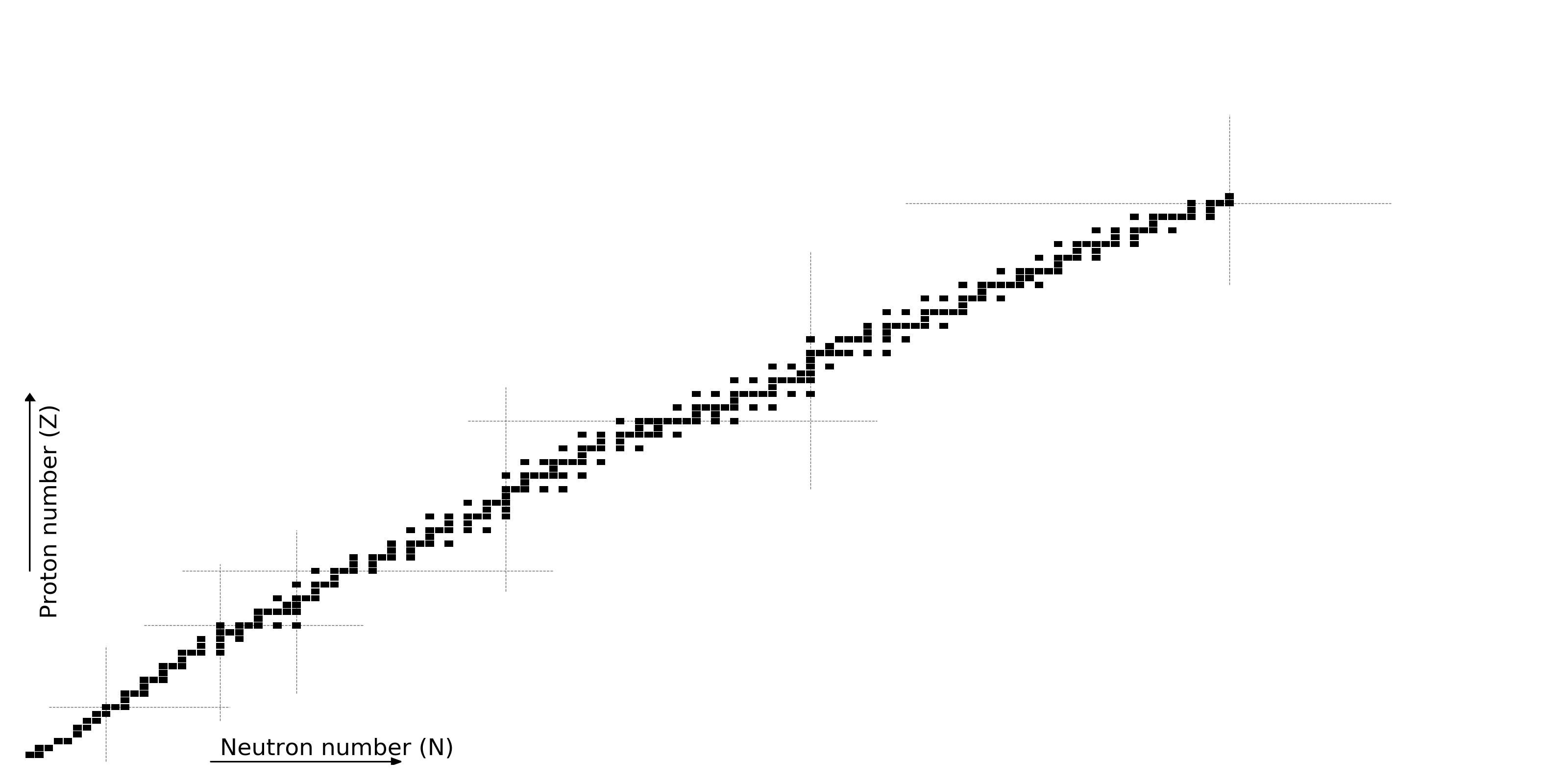Figure by Mumpower

## What do we know?

All half-lives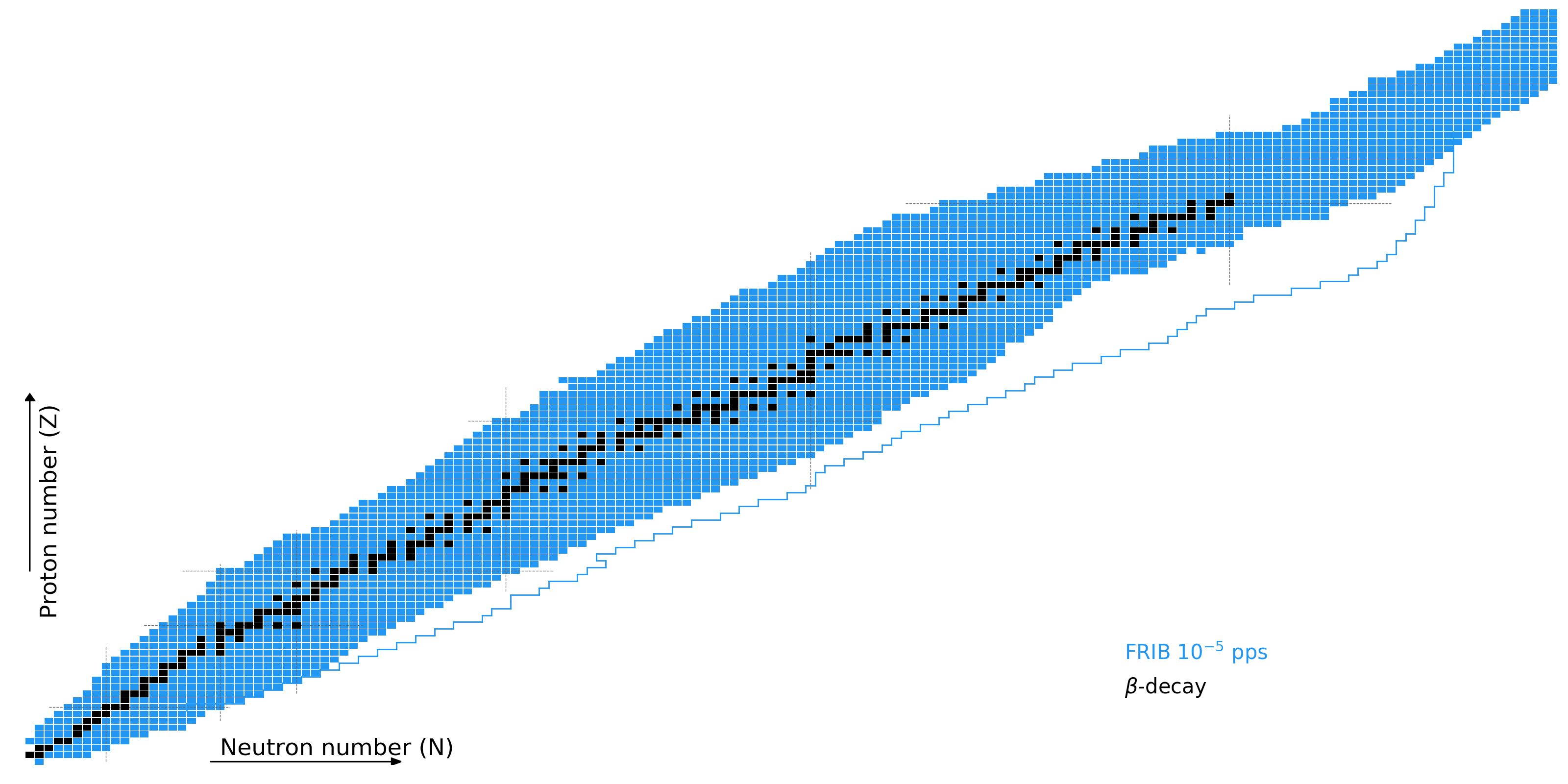Figure by Mumpower

## What do we know?

Nuclear masses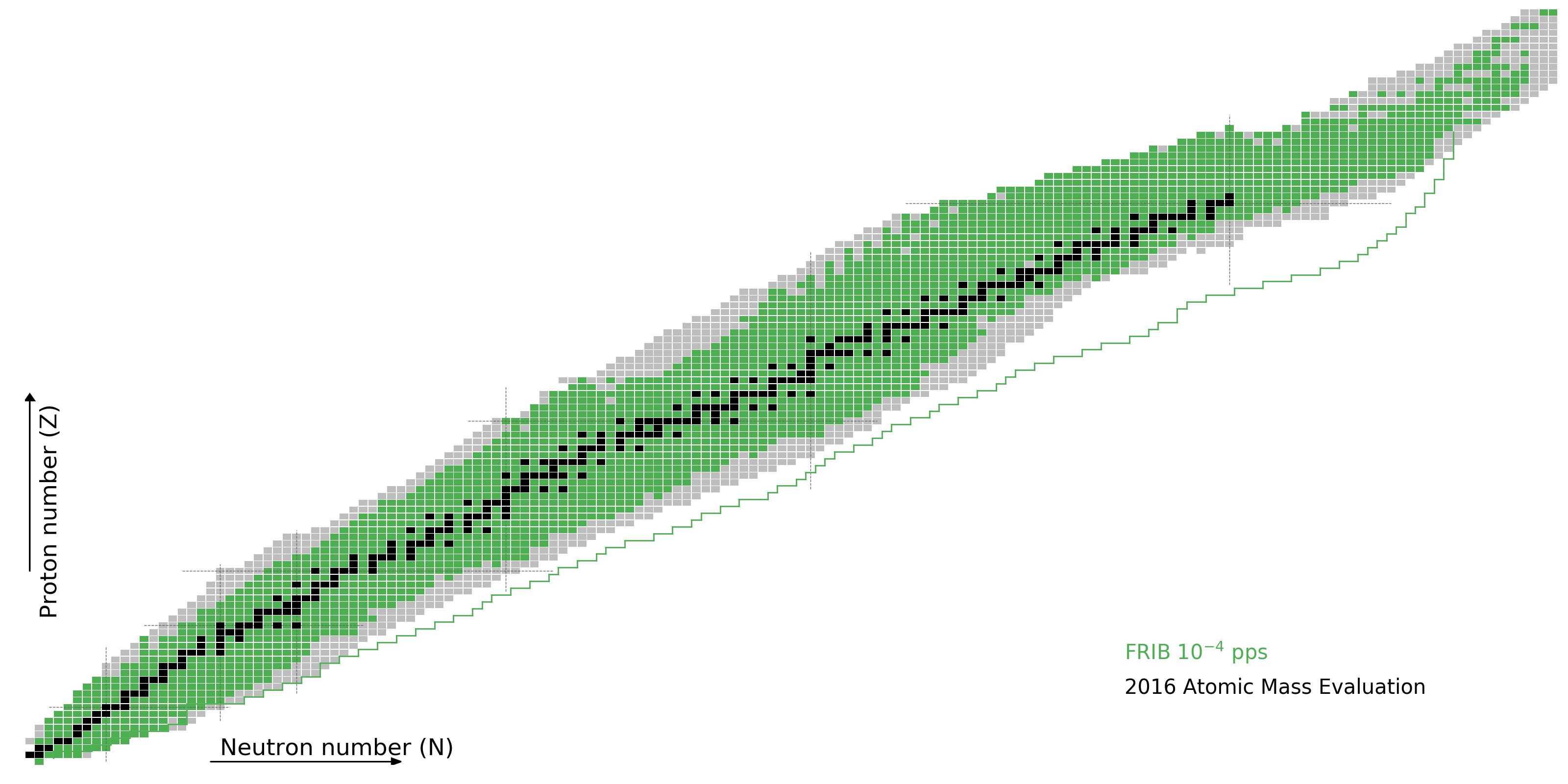Figure by Mumpower

## What do we know?

Neutron capture rates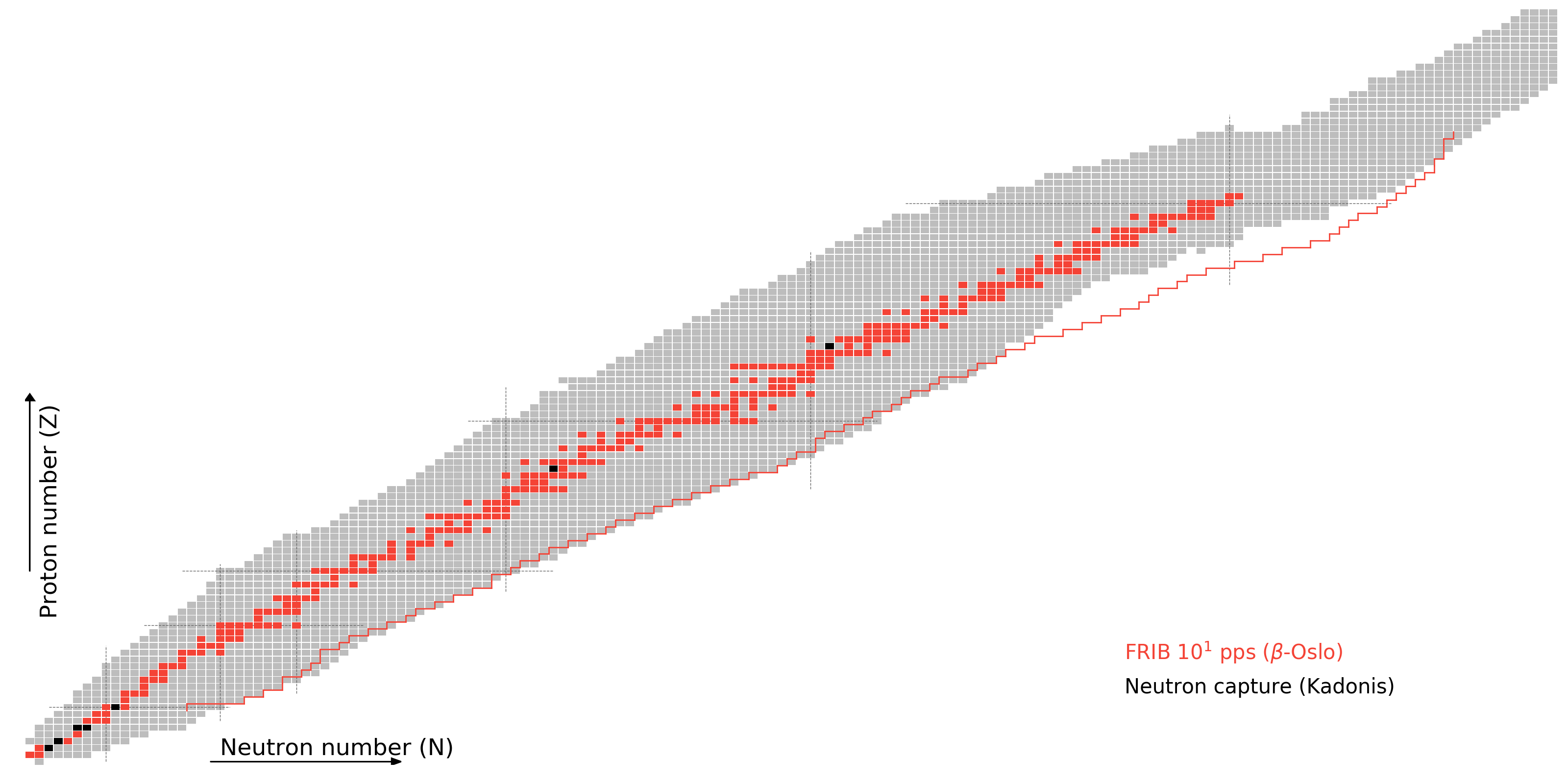Figure by Mumpower

## What do we know?

As of today, to varying degrees of accuracyFigure by Mumpower

## The Future

FRIB as the $r$-process machineFigure by Mumpower

## $r$-Process Calculation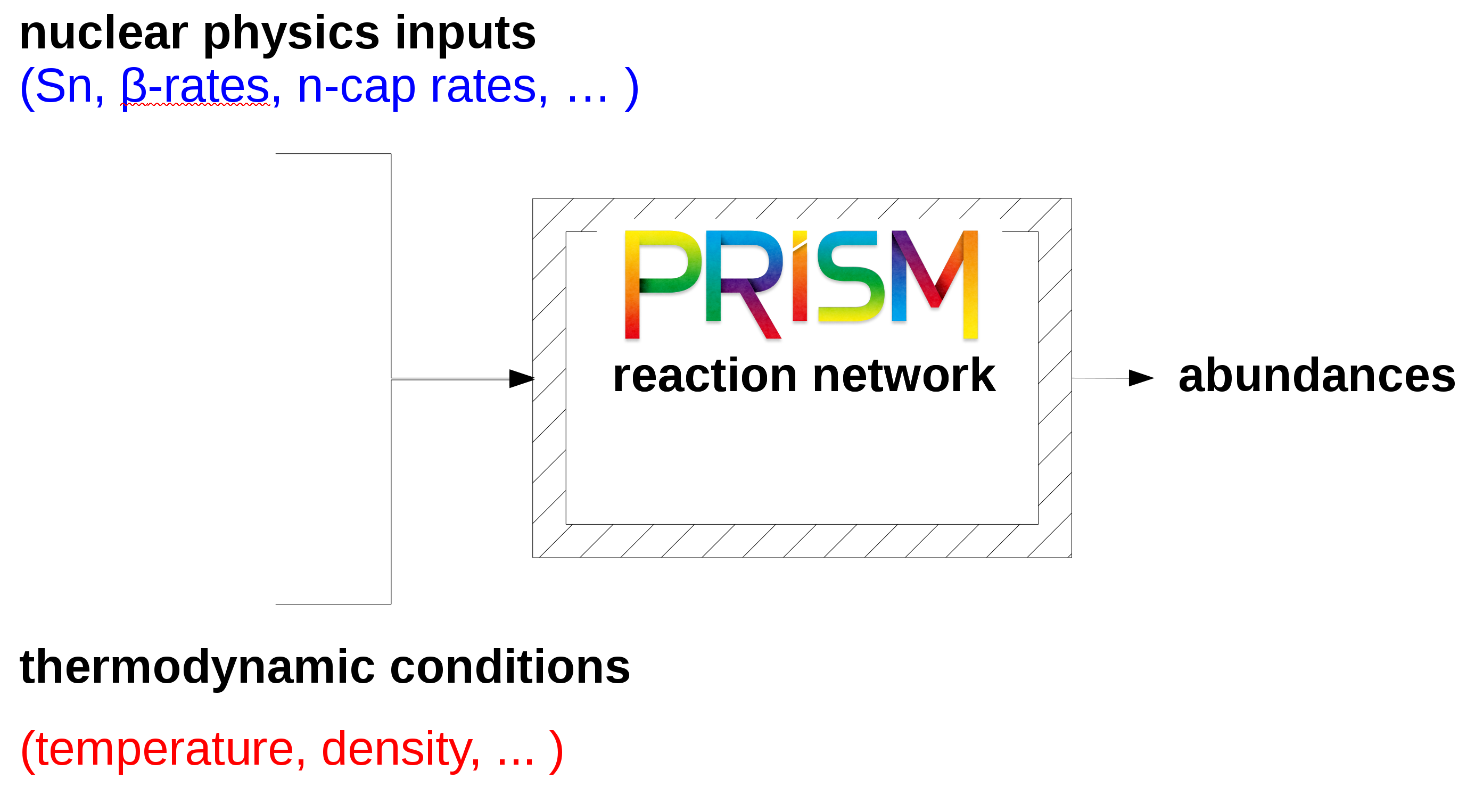PRISM: Portable Routines for Integrated nucleoSynthesis Modeling

Sprouse & Mumpower in prep (2019)

# Results

## Variation in masses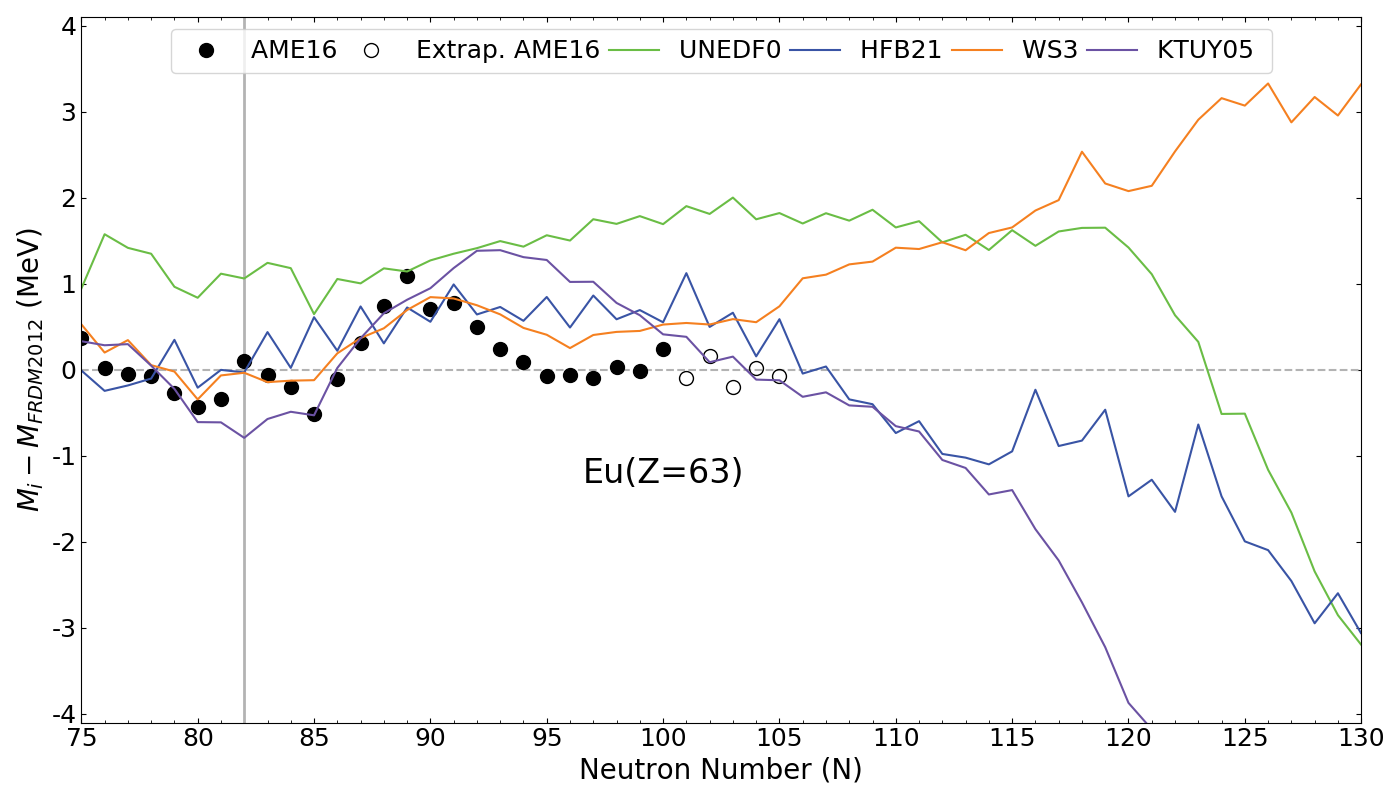Large variation in mass model predictions further from stability

Modified from Mumpower et al. PPNP 86 86-126 (2016)

## Why focus on masses?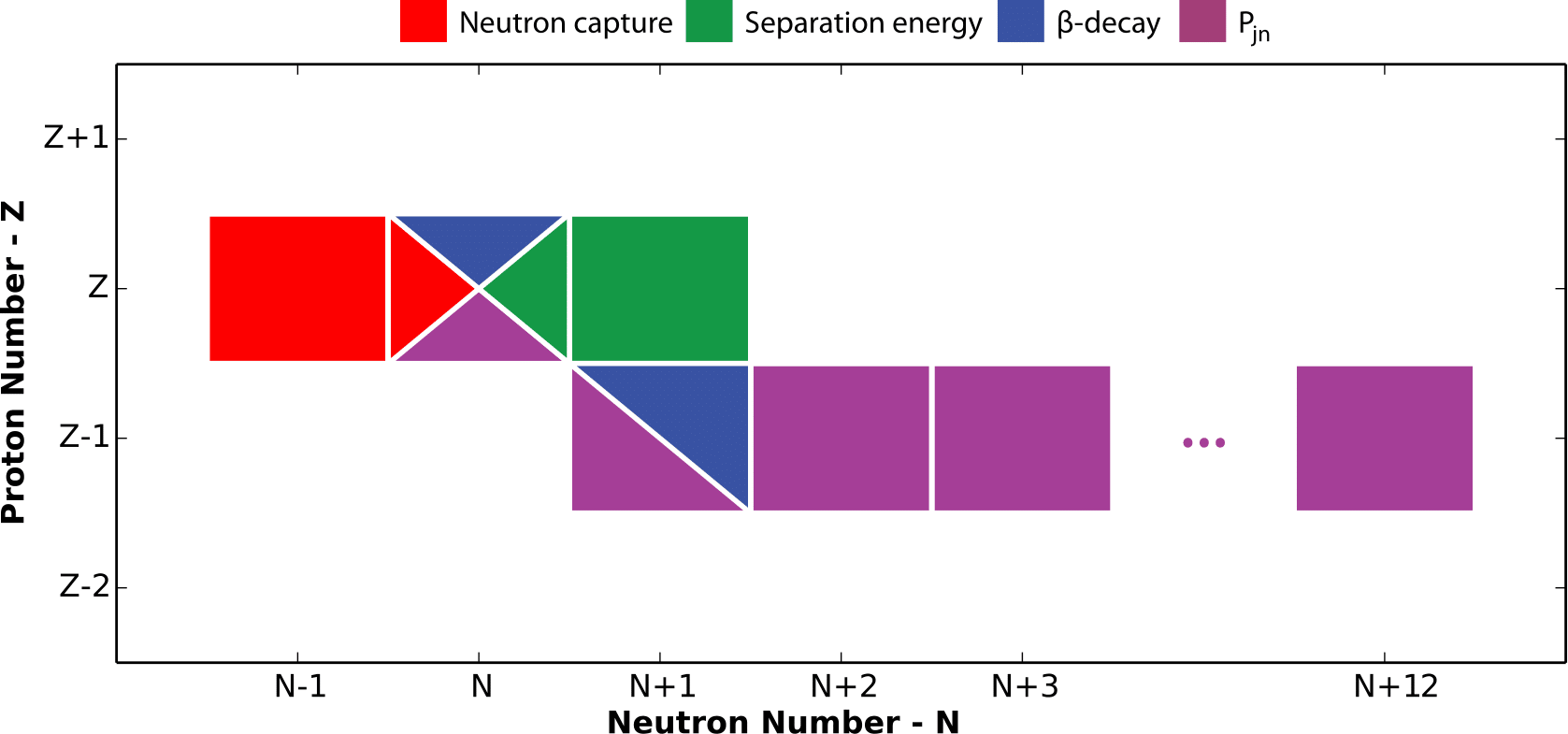Masses go into the calculation of all other relevant quantities...

Thus if we change a single mass in our network then we have to recalculate many other quantites!

Mumpower et al. PRC 92 035807 (2015)

## Uncertainties from masses

Hot wind: $S\sim200$, $\tau=80$ ms, $Y_e=0.3$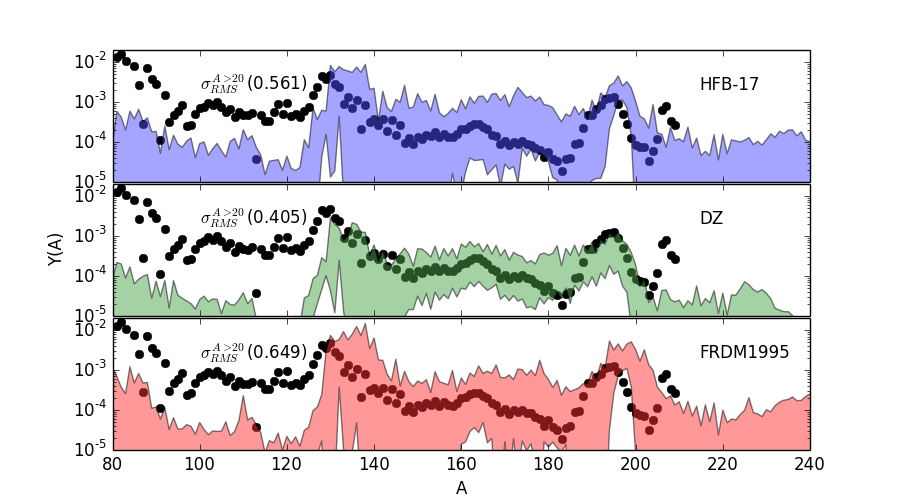Uncorrelated mass Monte Carlo study; not full propagation

Mumpower et al. PPNP 86 86-126 (2016)

## Uncertainties from masses

Hot wind: $S\sim200$, $\tau=80$ ms, $Y_e=0.3$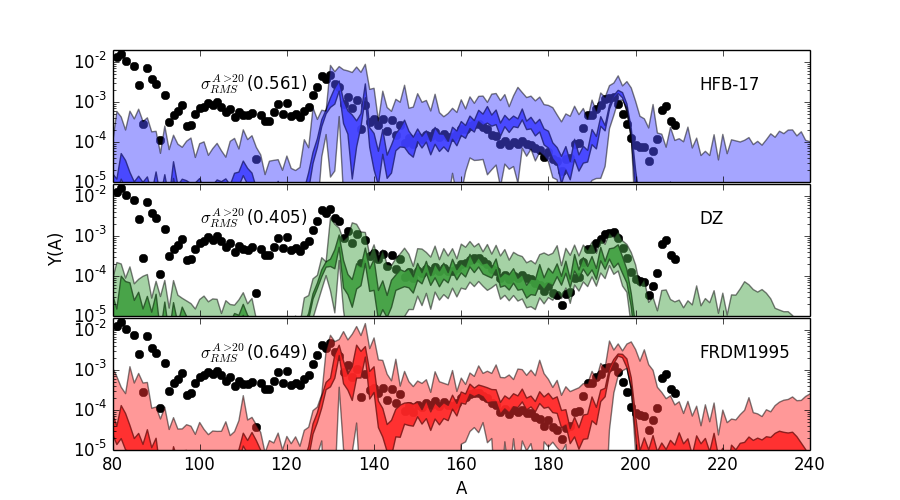Uncorrelated mass Monte Carlo study; not full propagation

Rule of thumb: $\Delta_\text{mass}\sim500$ keV $\Rightarrow$ $\Delta_Y\sim 2-3$ orders of magnitude

Mumpower et al. PPNP 86 86-126 (2016)

## The rare earth peak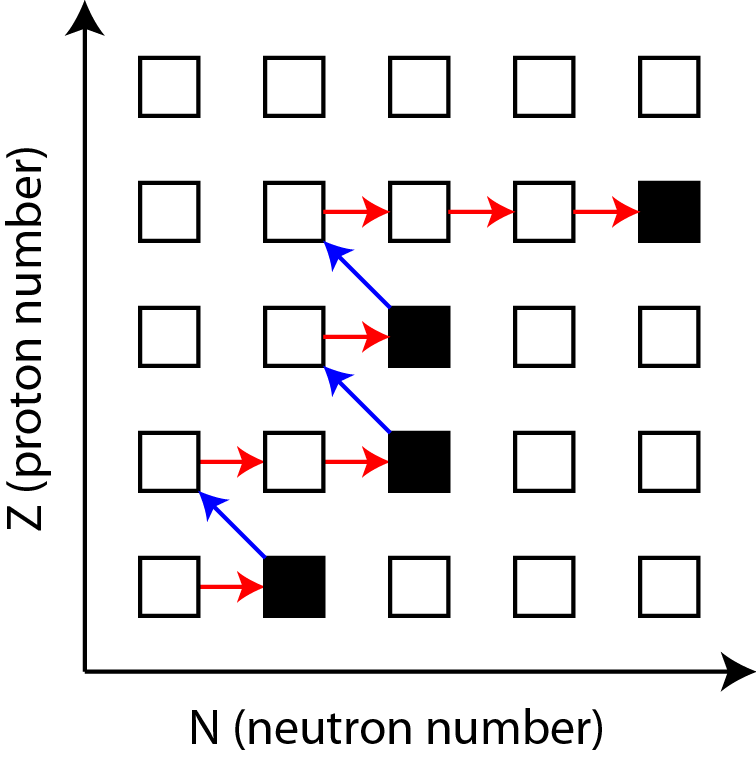Proposed ways to form the REP

1. Dynamical formation during freeze-out ($R\lesssim1$)
Requires a localized nuclear structure effect (kink)
2. Via fission fragment yields
Requires dumping heavy products in exactly the right spot

Region of enhanced lanthanide production (important for observations)

Sensitive to nuclear physics inputs and astrophysical conditions

Surman & Engel PRL 79 1809 (1997) • Mumpower et al. PRC 85 045801 (2012) • Mumpower et al. ApJ 752 117 (2012)

## The Bayesian Approach

An example... The Monty Hall problem

A new car is hidden behind one of the doors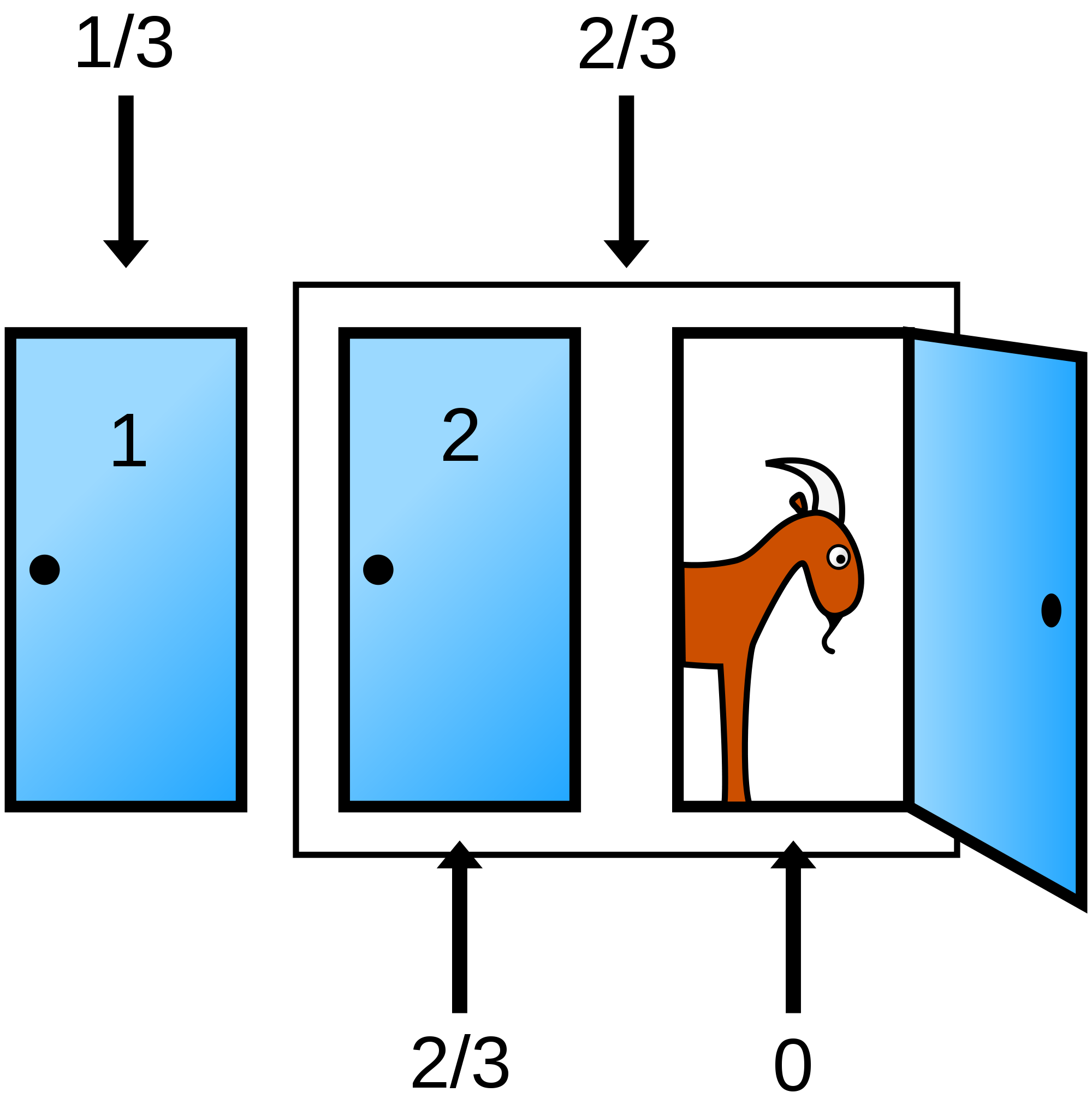The optimal strategy is to switch the initial pick - twice the chance of winning the new car

We update our probabilities based off new information

Reverse engineering: Update mass prediction based off the match of $r$-process calculation to observations

Mumpower et al. ApJ 833 282 (2016) • Mumpower et al. J. Phys. G 44 3 034003 (2017) • Vassh et al. in prep (2018)

## Our new mass predictions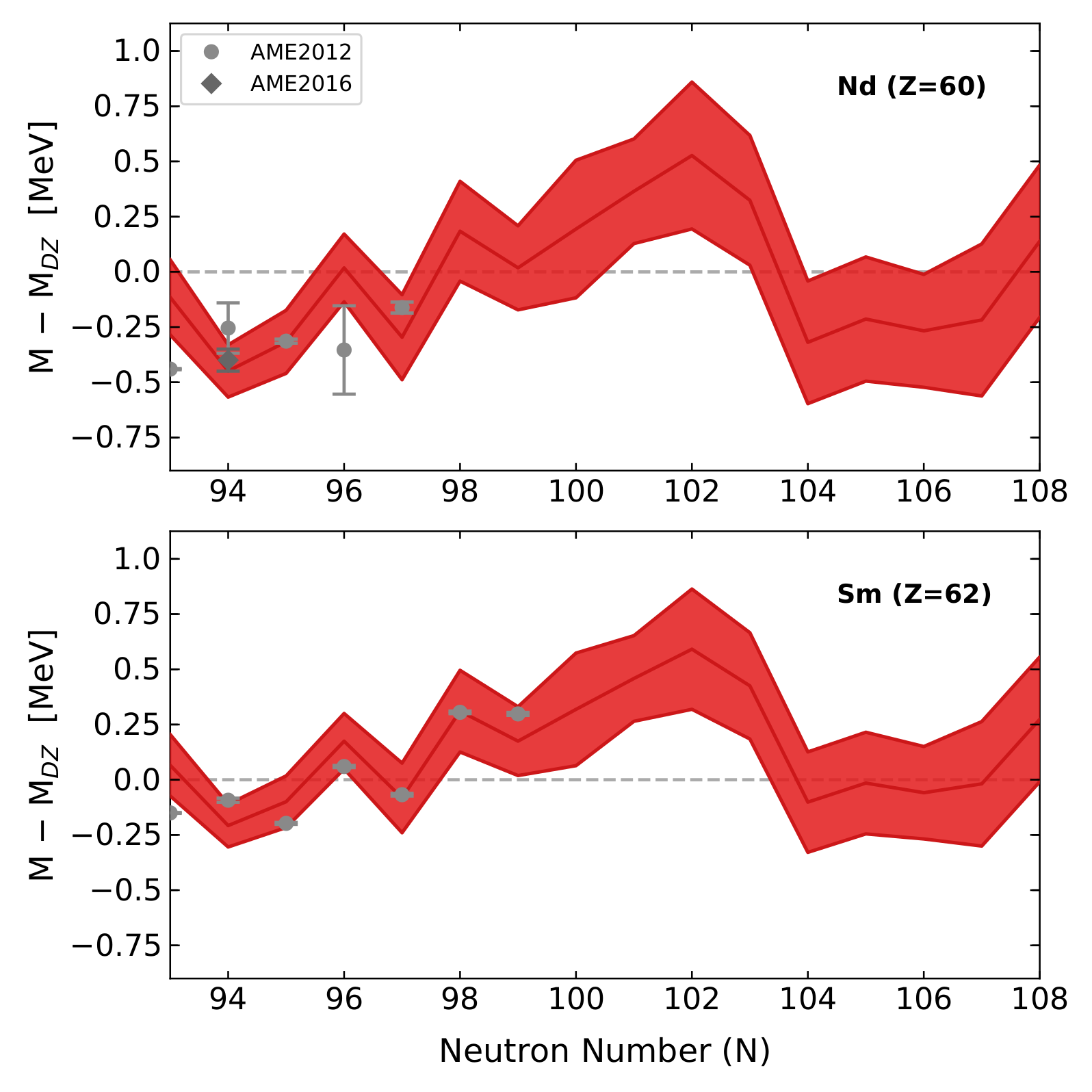Bayesian prior (DZ mass prediction) is a straight horizontal line

Our algorithm found a The trend

Orford et al. PRL 120 262702 (2018)

## Our new mass predictions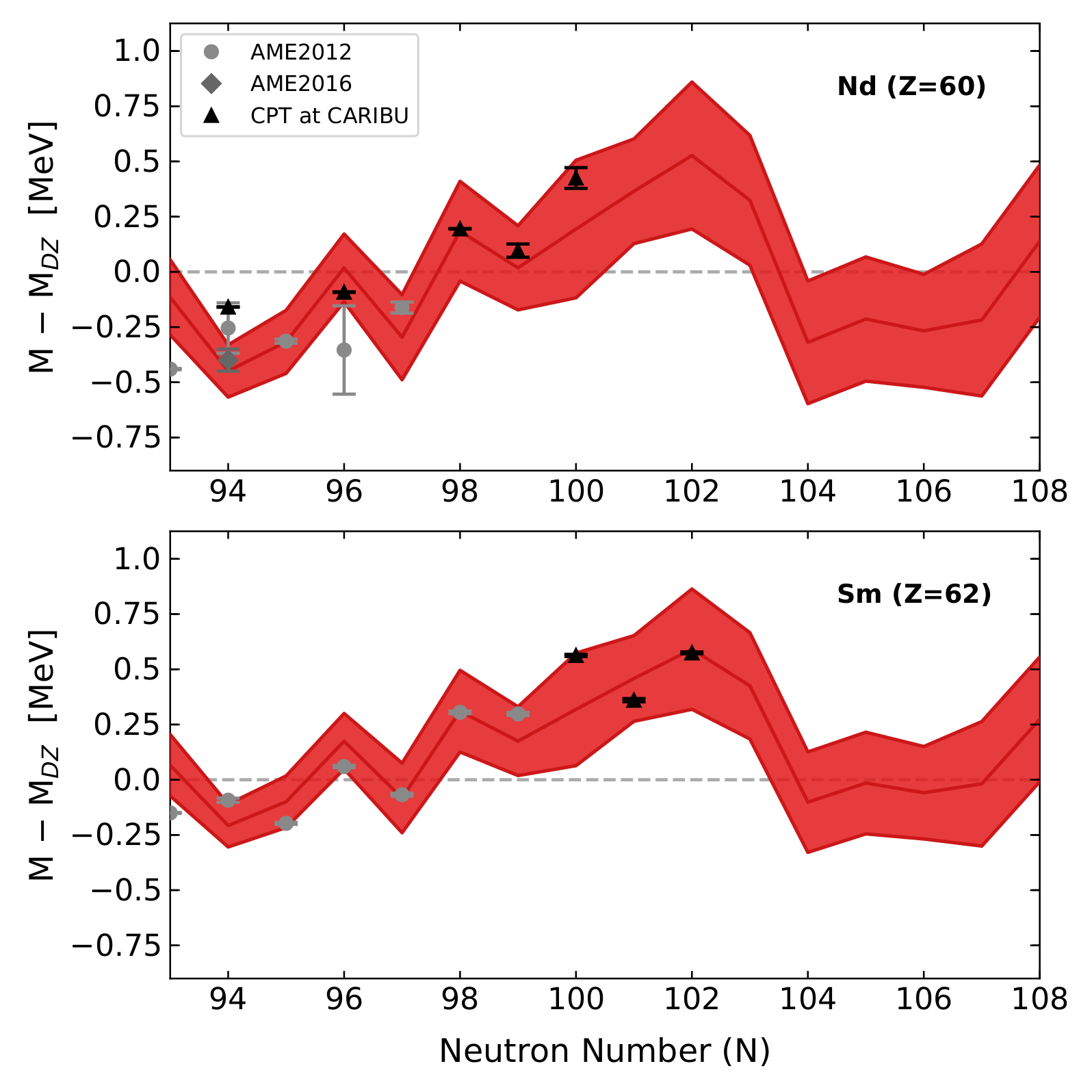The predicted trend matches CPT data!

The downturn between N=102 and N=104 is near the midshell - critical for peak formation - will it also be found?

Orford et al. PRL 120 262702 (2018)

## Sensitivity to astrophysical conditions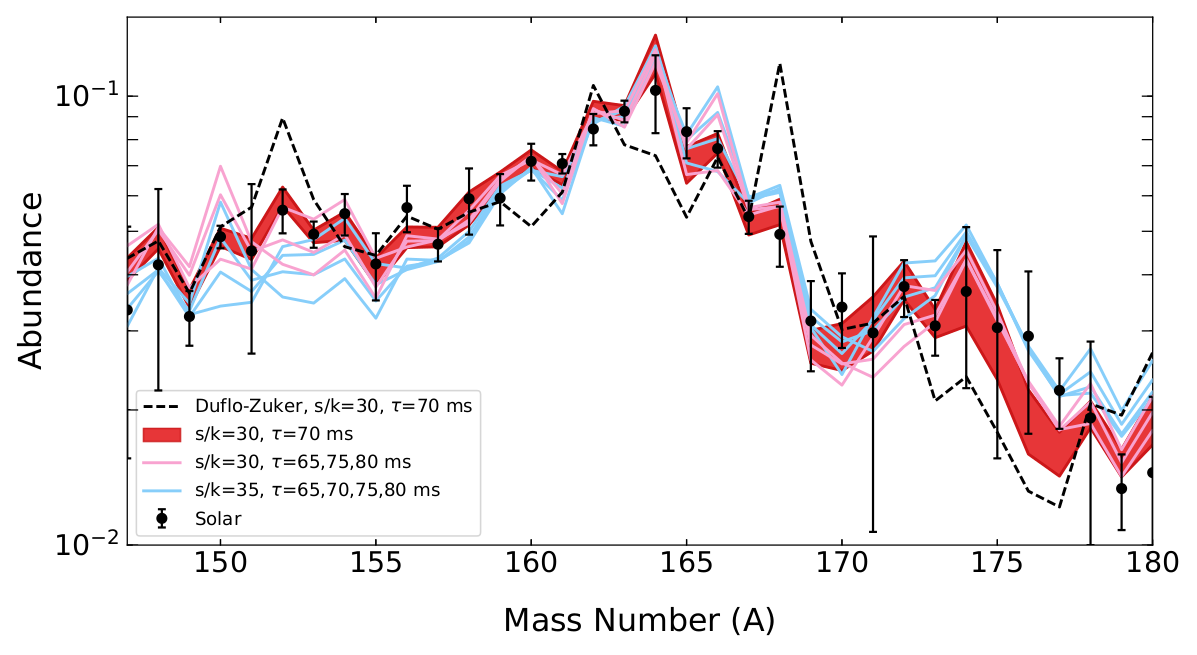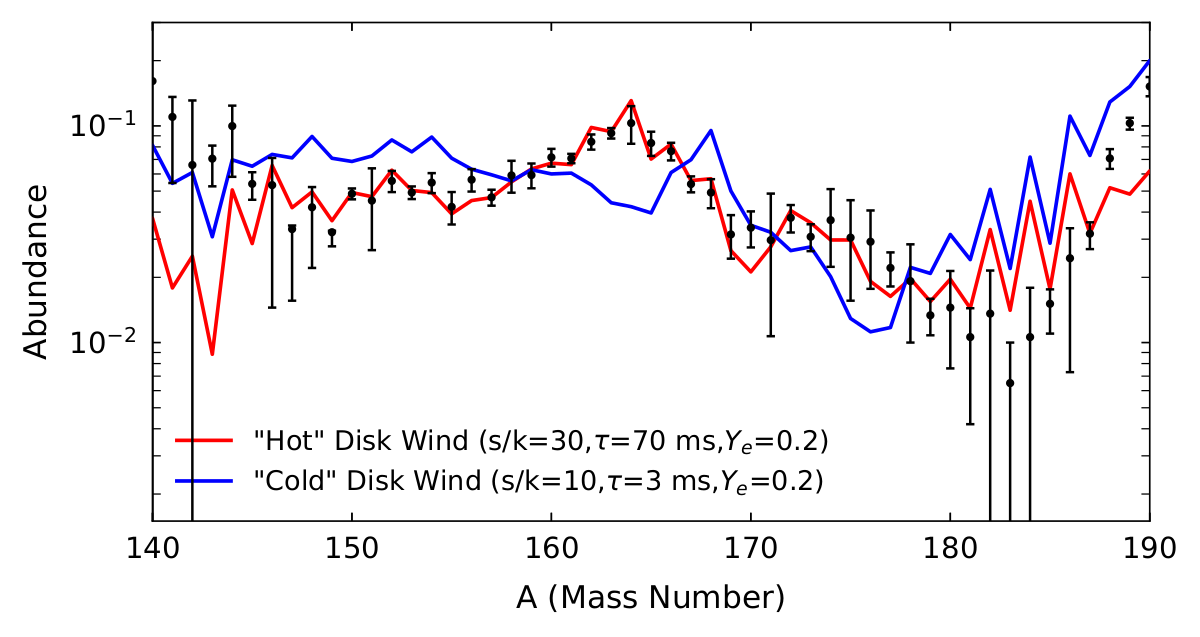The trend is robust for small changes to astrophysical conditions (left)

But if we change the conditions too much (right) we no longer fit the abundances

This result is completely dependent on the N=104 feature which has yet to be measured

The possibility for mass measurements to discrimenate between astrophysical conditions is nearly at hand!

Orford et al. PRL 120 262702 (2018) • Vassh et al. in prep (2018)

## $\beta$ -delayed neutron emission

Discovered in 1939 by R.B. Roberts et al.

Delayed emission with half-life of precursor

Energetically possible: $Q_\beta$ > $S_n$ Important for neutron-rich nuclei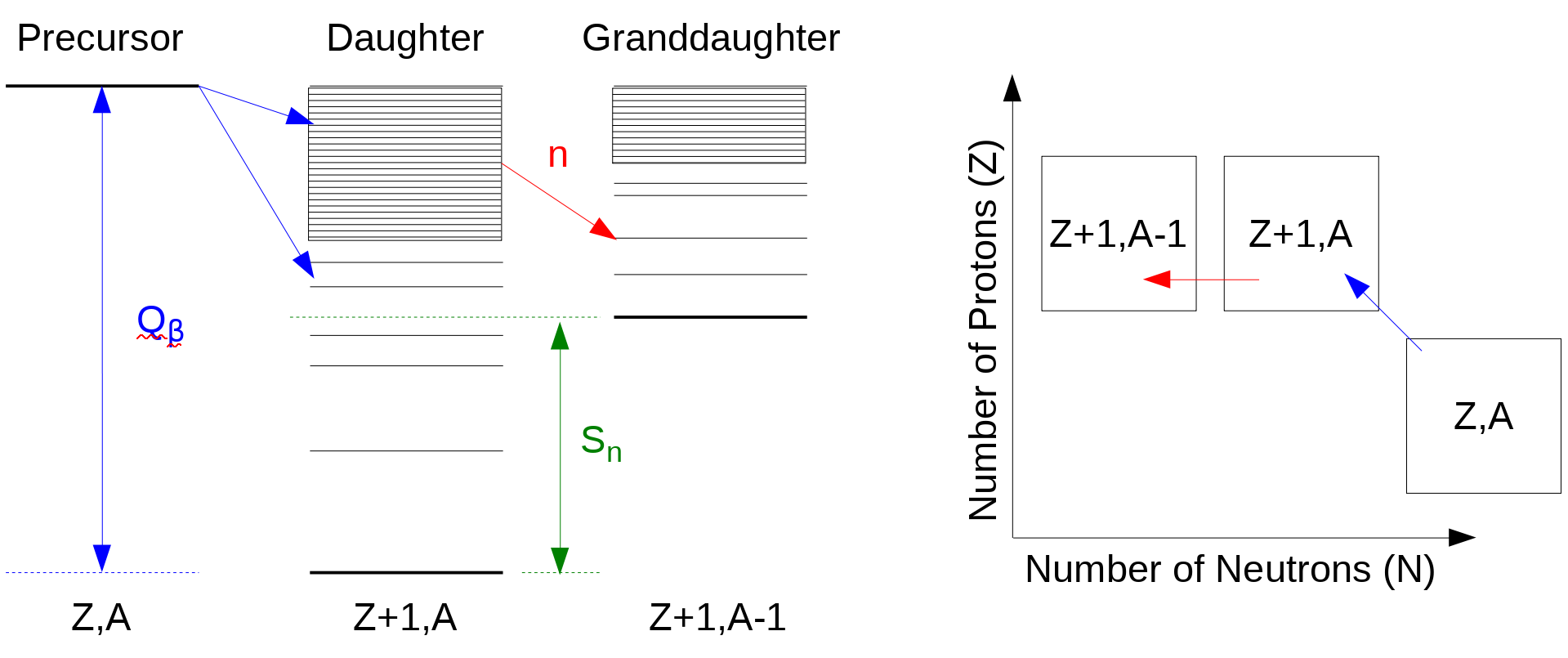## Combining QRPA + HF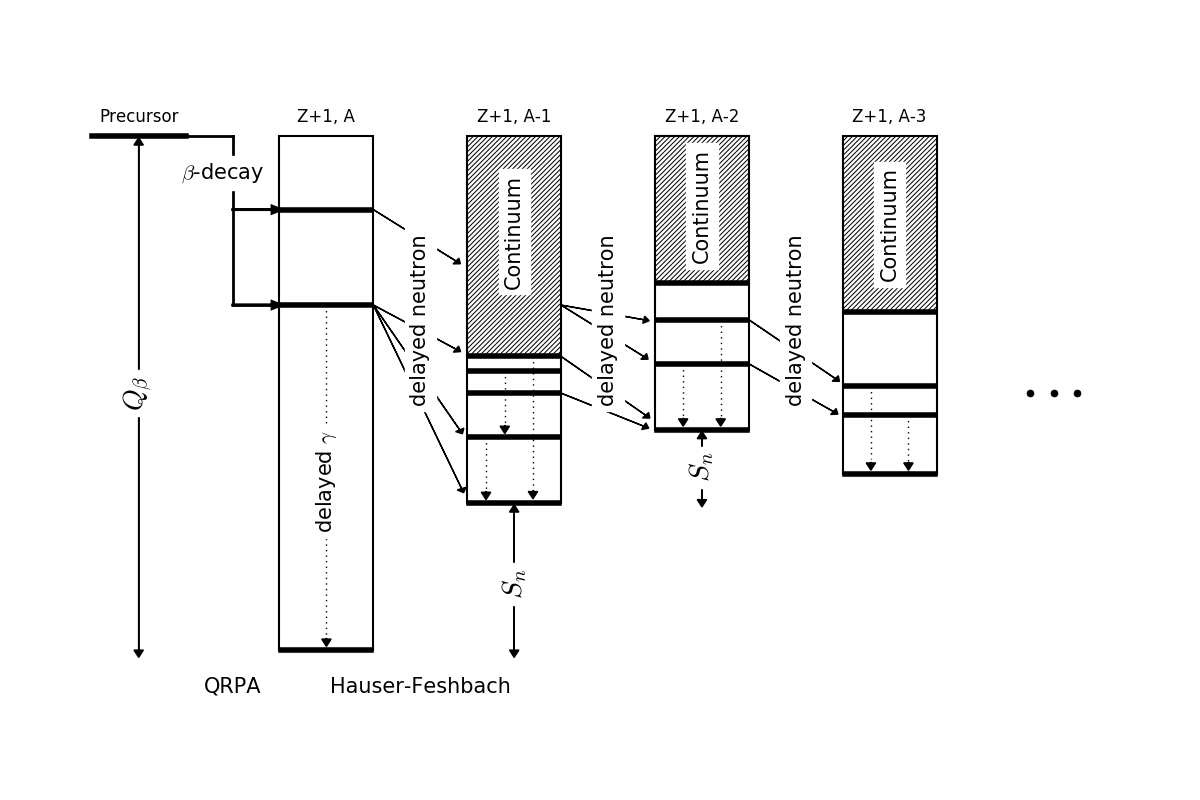Initial population from the $\beta$-decay strength function from P. Möller's QRPA

Follow the statistical decay until all excitation energy is exhausted

Möller et al. PRC (1997 & 2003) • Kawano et al. PRC 94 014612 (2016) • Mumpower et al. PRC 94 064317 (2016)

## Average neutron emission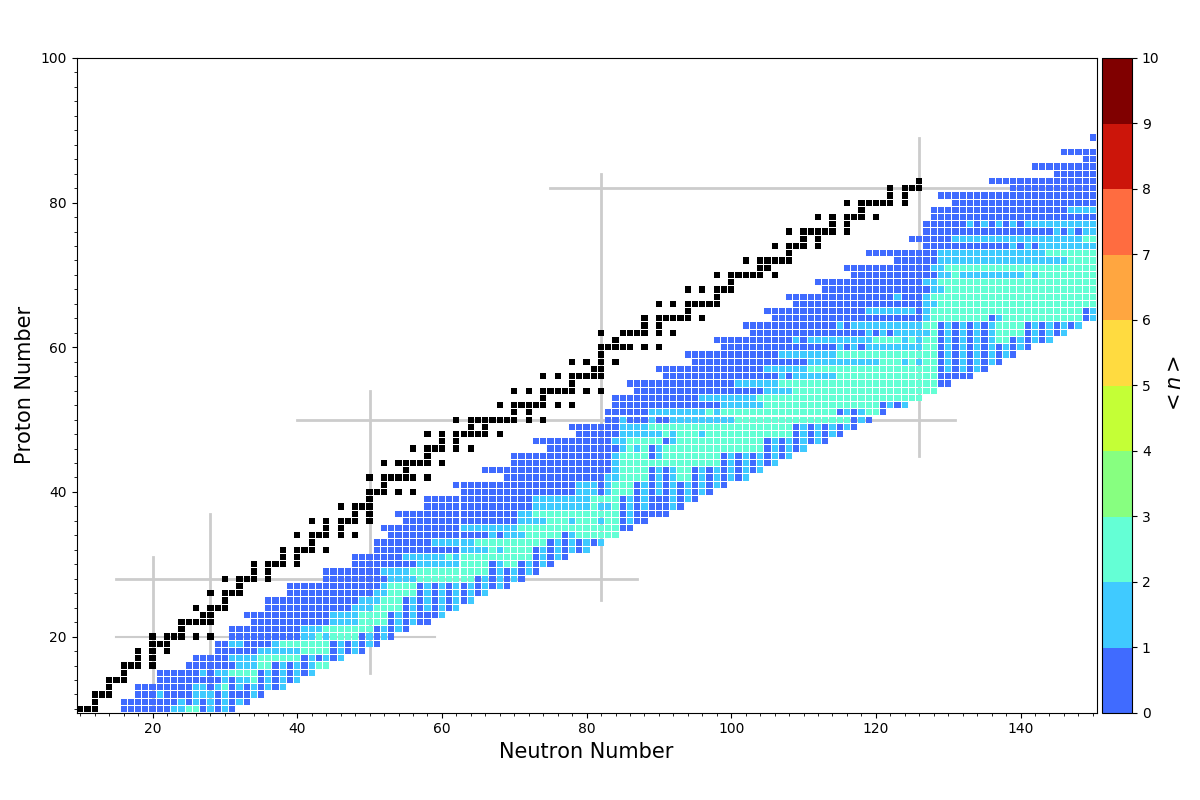Apply energy window method to the entire chart of nuclides

Problem with describing very neutron-rich nuclei

Mumpower et al. PRC 94 064317 (2016)

## Average neutron emission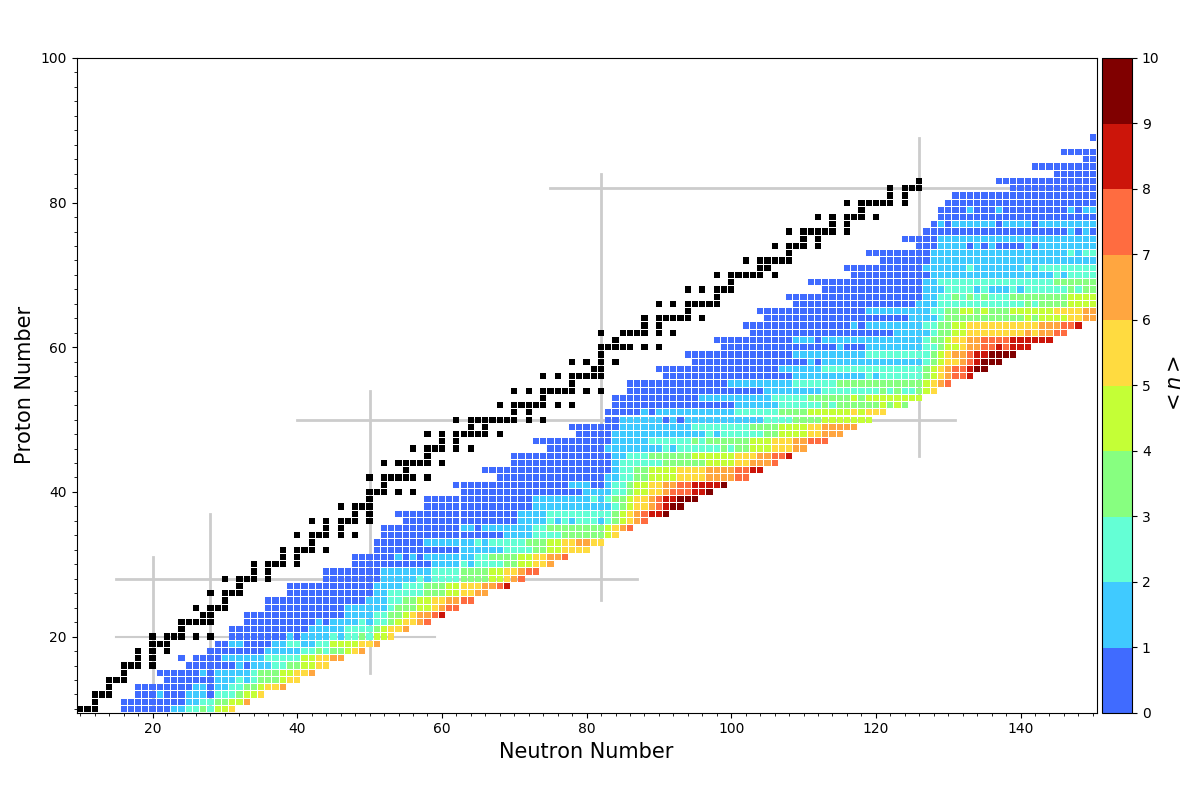Apply the QRPA+HF method to the entire chart of nuclides

Problem with neutron-rich nuclei goes away

Mumpower et al. PRC 94 064317 (2016) • Möller et al. ADNDT (2018)

## Extensive benchmarking

QRPA+HF GT-only $\beta$-strength are within 15% of measured $P_{1n}$ values

Adding FF transitions improves the match to measured data by 3%

Using measured masses improves the match to measured data by 3%

This yields a roughly 9% global model uncertainty to measured $P_{1n}$ values

Can we extend this model to other phenomena ... fission?

Spyrou et al. PRL 117 142701 (2016) • Mumpower et al. PRC 94 064317 (2016) • Wu et al. PRL 118, 072701 (2017)

## Extension to $\beta$ df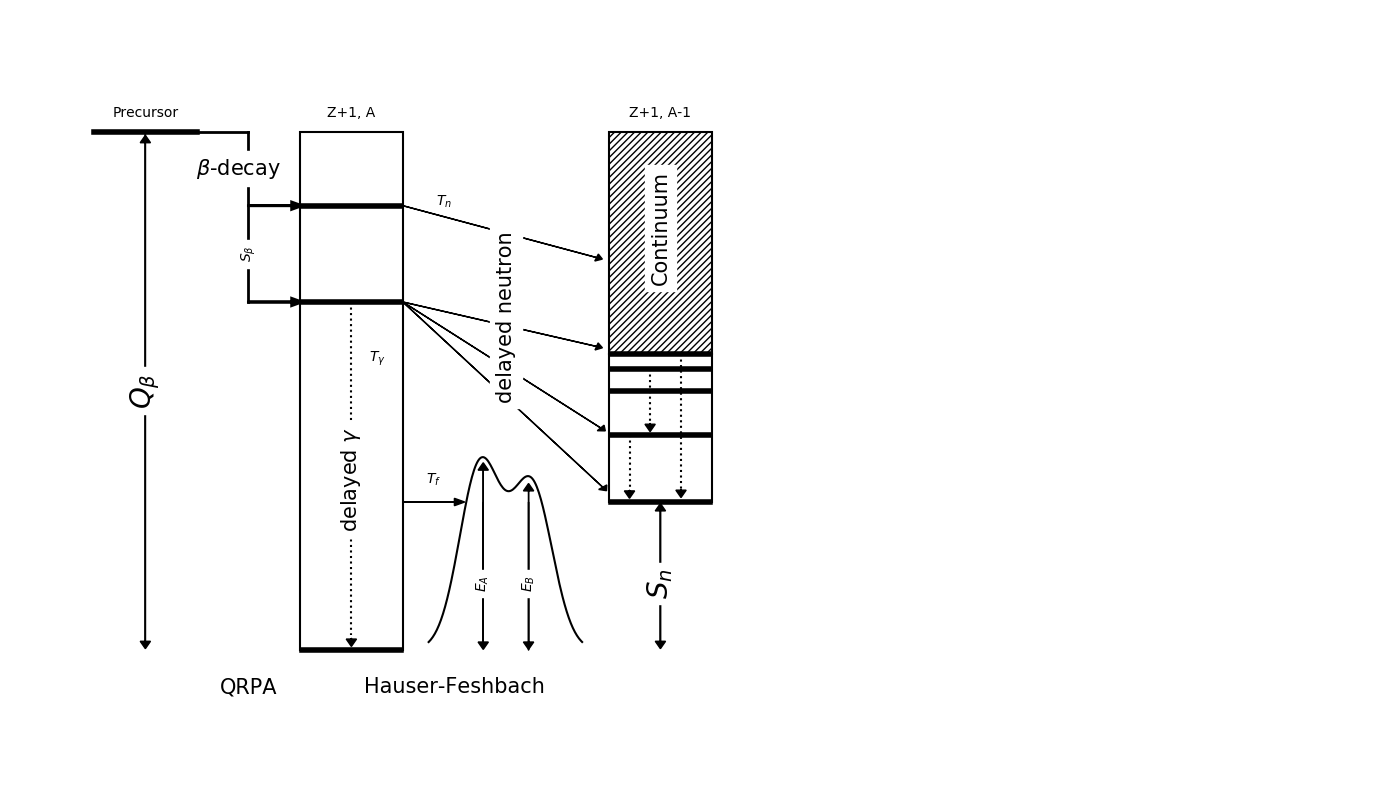We have recently extended the model to describe $\beta$-delayed fission ($\beta$df)

Barrier heights from Möller et al. PRC 91 024310 (2015)

Assumes a Hill-Wheeler form for fission transmission

Mumpower et al. arXiv:1802.04398 Accepted ApJ (2018)

## Multi-chance $\beta$ dfRecall: Near the dripline $Q_{beta}$ ⇡ $S_{n}$ ⇣

Multi-chance $\beta$df: each daughter may fission

The yields in this decay mode are a convolution of many fission yields!

Mumpower et al. arXiv:1802.04398 Accepted ApJ (2018)

## ($n$,$\gamma$,$f$) competition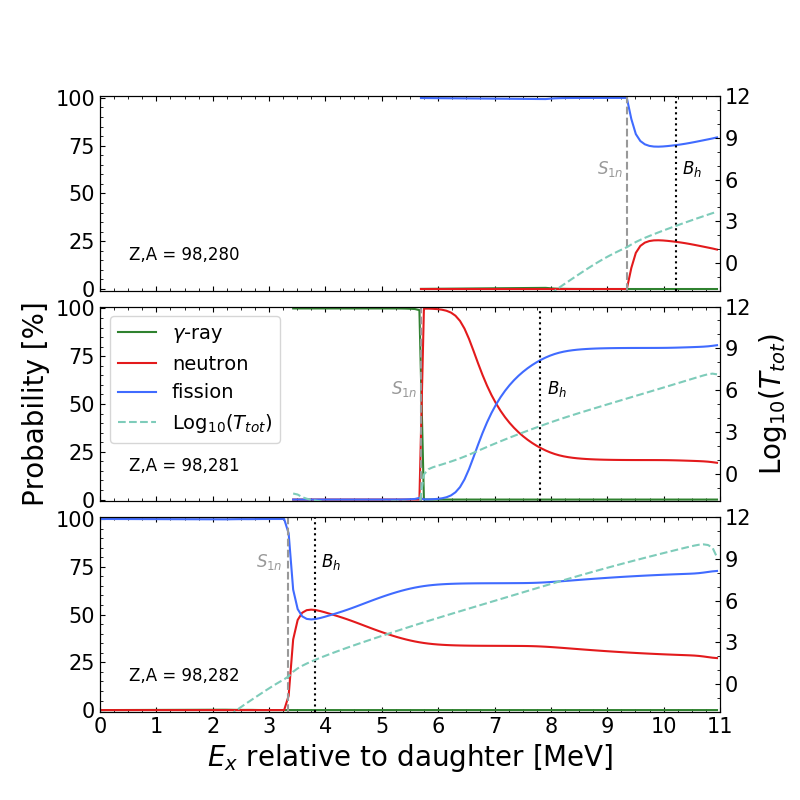Fission can successfully compete with $\gamma$-rays and neutrons

Mumpower et al. arXiv:1802.04398 Accepted ApJ (2018)

## Cumulative $\beta$ df probability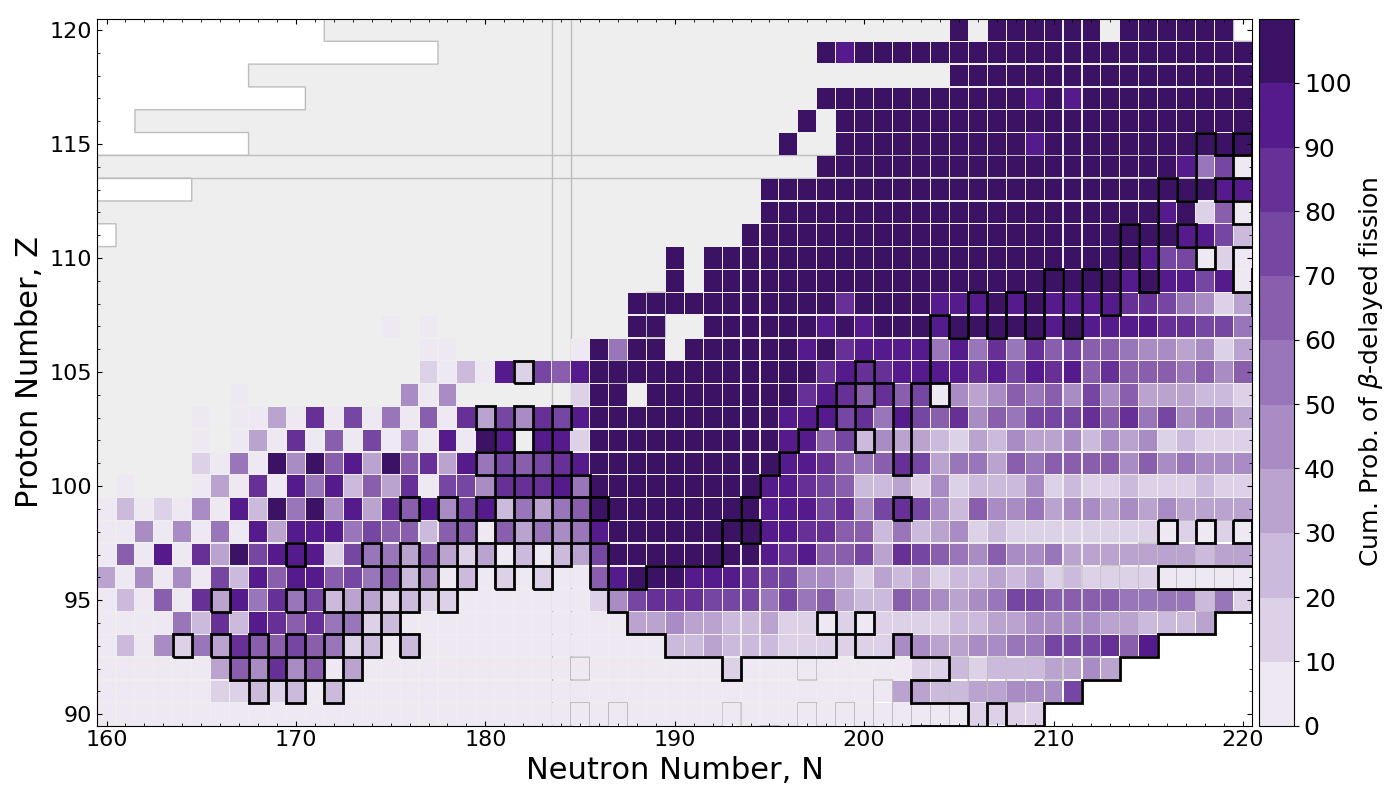$\beta$df occupies a large amount of real estate in the NZ-plane

Multi-chance $\beta$df outlined in black

Mumpower et al. arXiv:1802.04398 Accepted ApJ (2018)

## Application to the $r$-process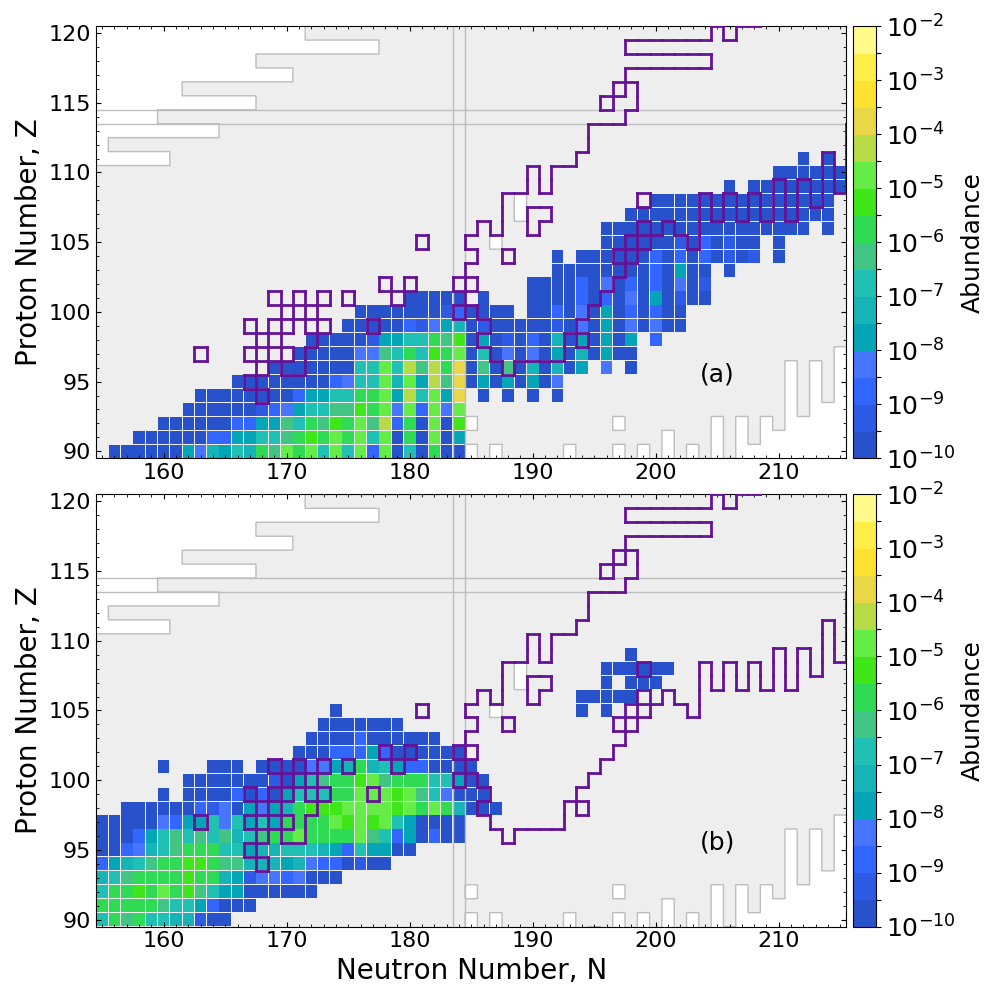Network calculation of neutron star merger ejecta

$\beta$df alone prevents the production of superheavy elements in nature

Mumpower et al. arXiv:1802.04398 Accepted ApJ (2018)

## The N=126 Factory

Probe nuclei FRIB may have trouble producing...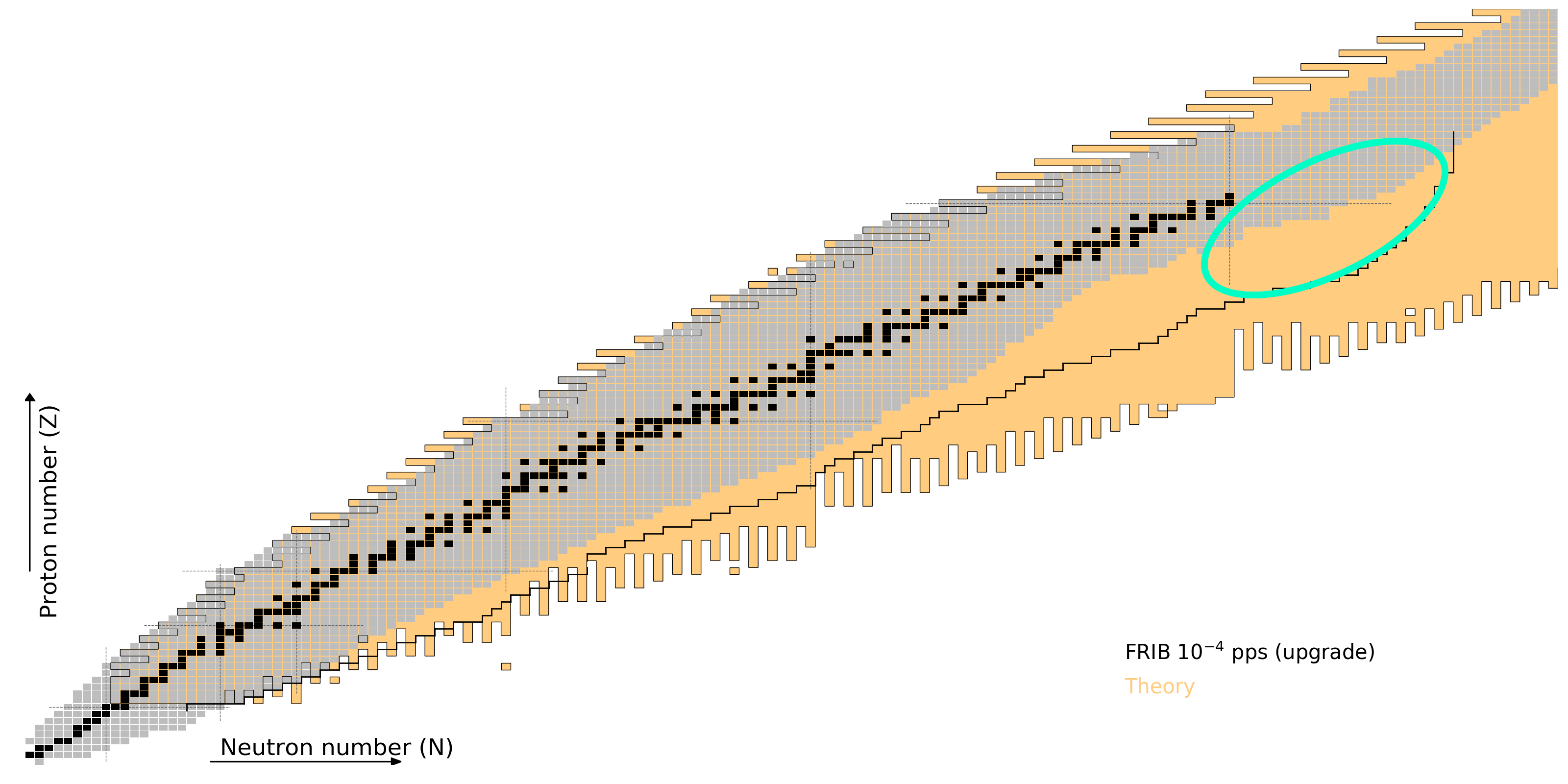Figure by Mumpower

## Importance for the $r$-process

A dirty secret: nucleosynthesis simulations have trouble reproducing
(1) the peak height and (2) the position of the A=195 peak (due to the N=126 shell closure)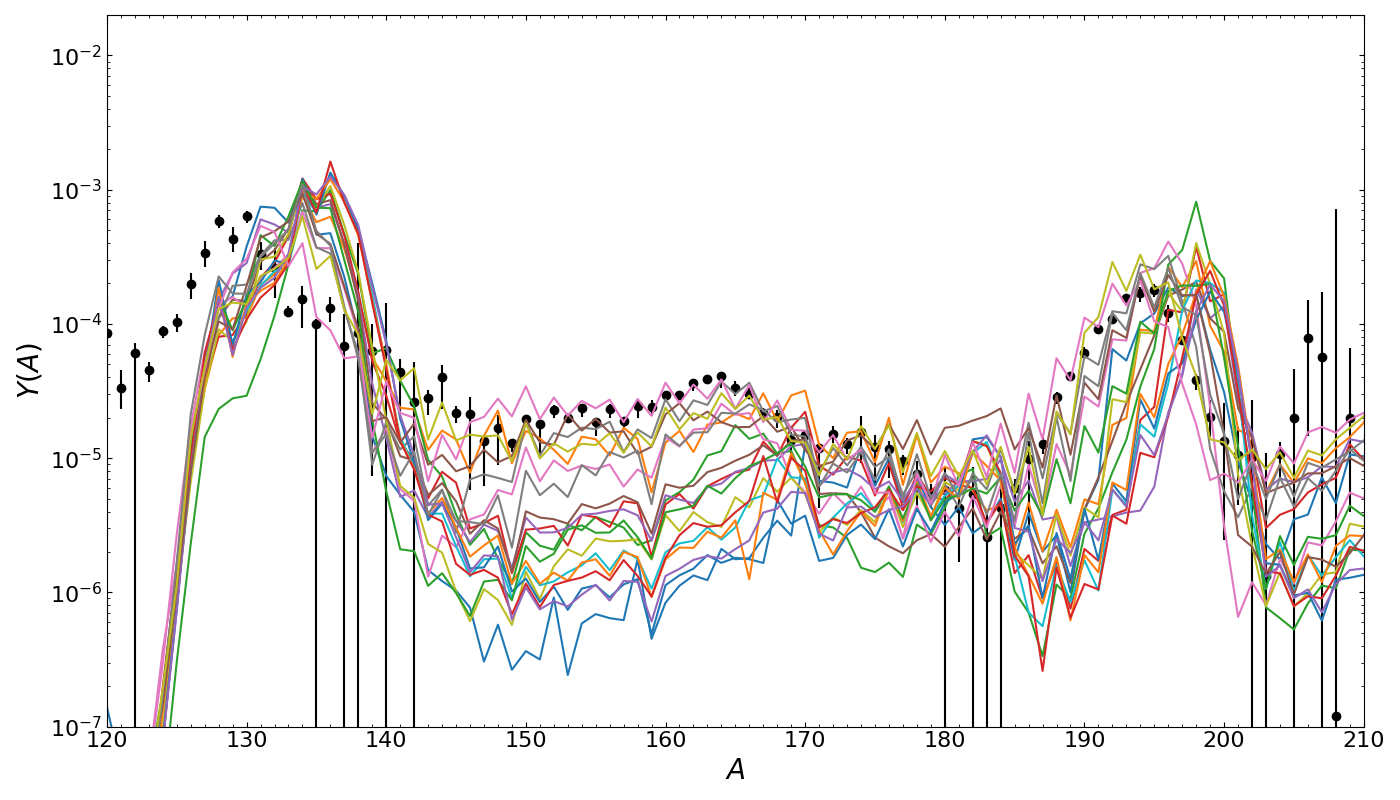Final abundances using 20 mass models given the same astro. conditions

The N=126 factory can help us to understand the evolution of shell structure, GT vs FF contributions and will greatly impact $r$-process calculations

The N=126 shell closure acts as the gatekeeper to fission recycling

Calculation by Mumpower

## Calculated yieldJaffke et al. PRC 67 034608 (2018) • Y. Zhu et al. ApJL 863 2 (2018) • Mumpower et al. in prep. (2019)

## Observational Impact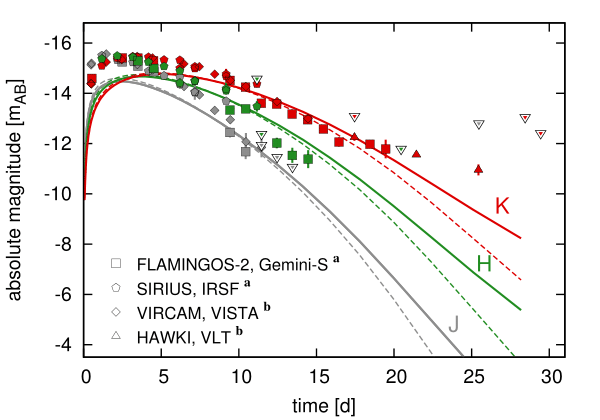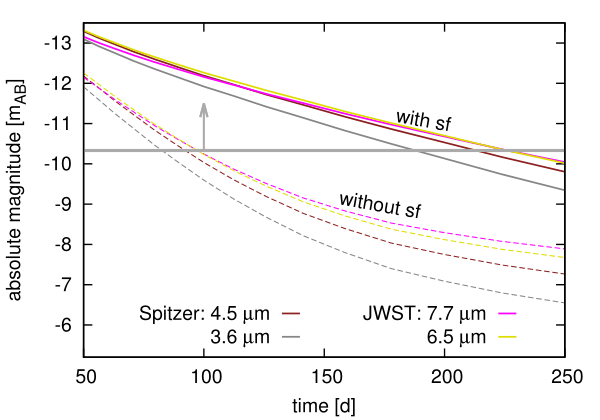Both near- and middle- IR are impacted by the presence of $^{254}$Cf

Late-time epoch brightness can be used as a proxy for actinide nucleosynthesis

Future JWST will be detectable out to 250 days with the presence of $^{254}$Cf

This is all directly tied to the strength of the N=126 shell closure!

Y. Zhu et al. ApJL 863 2 (2018)

## Special thanks to

My collaborators

A. Aprahamian, J. Clark, E. Holmbeck, P. Jaffke, T. Kawano, O. Korobkin, S. Liddick, G. C. McLaughlin, P. Möller, R. Orford, J. Randrup, G. Savard, A. Sierk, T. Sprouse, A. Spyrou, R. Surman, N. Vassh, M. Verriere, J. Wu, Y. Zhu
& many more...

Students Postdocs

## Summary

The binary neutron star merger event, GW170817, was recently observed using both gravitational waves and electromagnetic signals

It has answered some questions while opening others...

Robust models of nuclear physics are required to understand the abundance and electromagnetic signatures from the heavy element nucleosynthesis that may ensue from such events

Astrophysics can be used as an alternative benchmark for nuclear models, in addition to experimental data / evaluations

An effort to improve nuclear modeing is ongoing at LANL

Collaboration between Argonne & Los Alamos has been very successful with many projects to come!

Results at MatthewMumpower.com

# Extra Slides

## Actinide Boost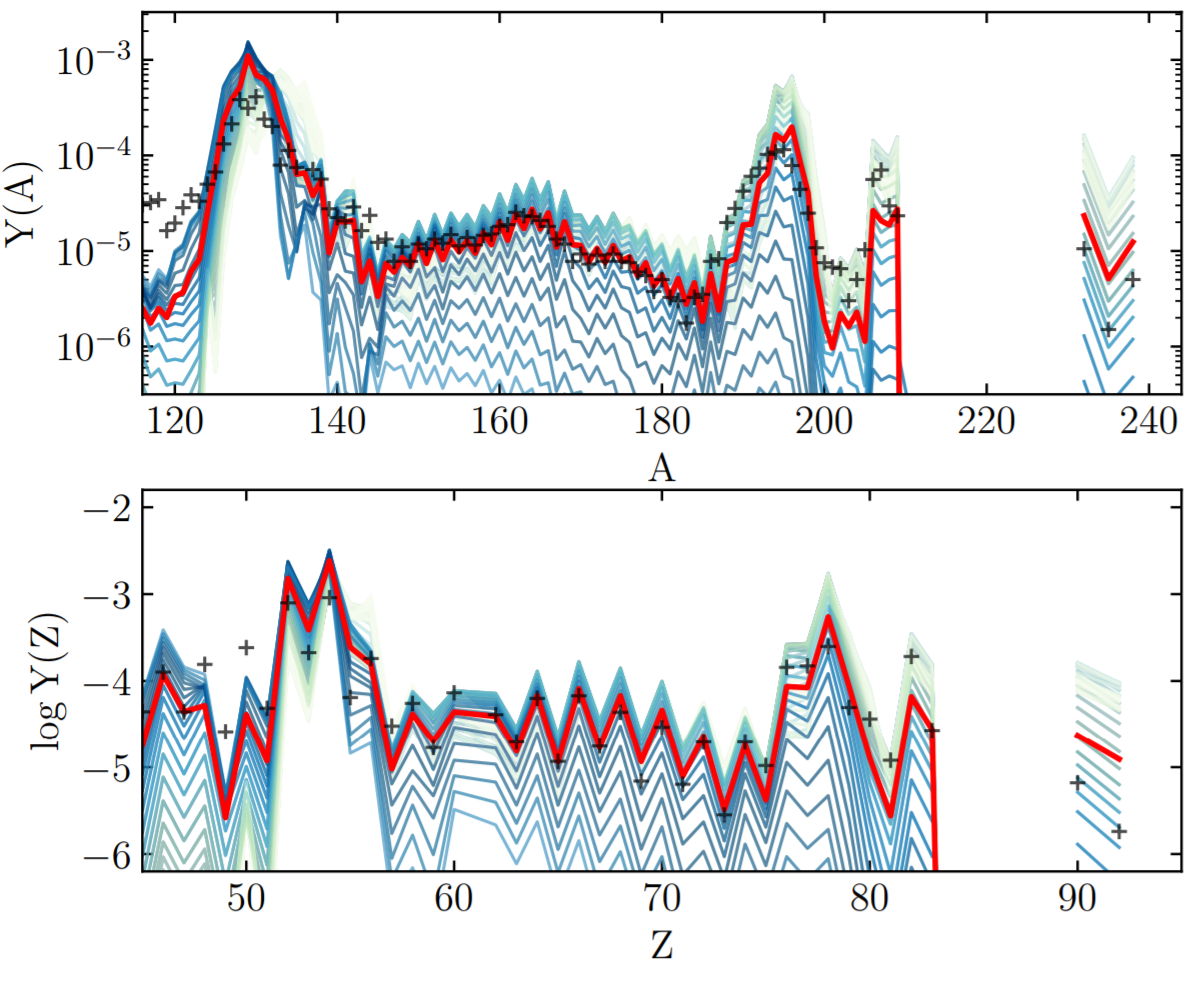If actinides are produced, they are always overproduced versus lanthanides / rare earth elements

A sufficient amount of dilution with ligher $r$-process material is required to match the solar isotopic residuals over a range of Z & A

Holmbeck et al. arXiv:1807.06662 [submitted ApJ] (2018)

## Impact on final abundances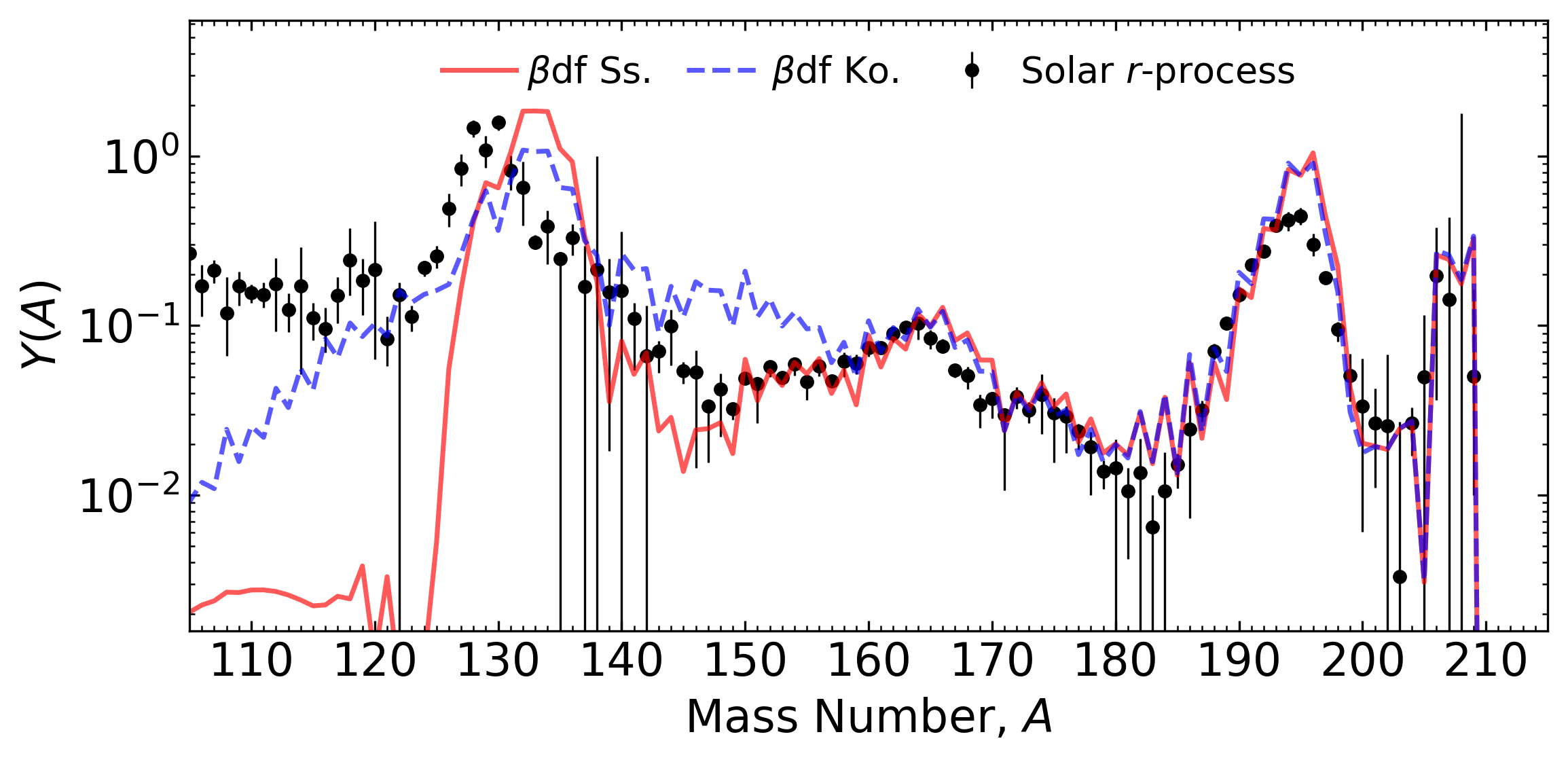Network calculation of neutron star merger ejecta; FRDM2012 inputs

$\beta$df can shape the final pattern near the $A=130$ peak

Mumpower et al. arXiv:1802.04398 Accepted ApJ (2018)

## Multi-chance $\beta$ df contribution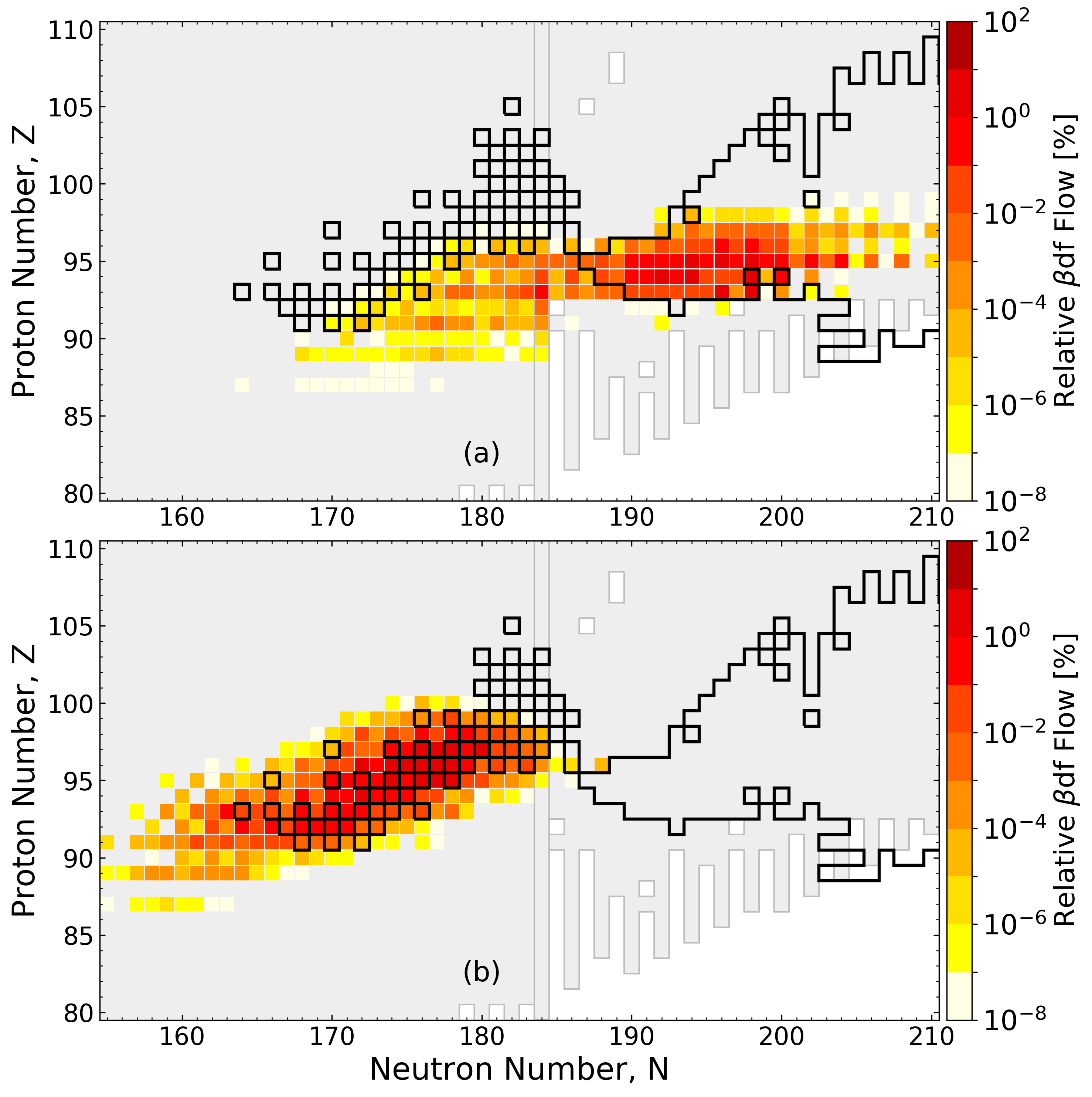Network calculation of neutron star merger ejecta; FRDM2012 inputs

Multi-chance $\beta$df contributes at both early and late times

Mumpower et al. arXiv:1802.04398 Accepted ApJ (2018)